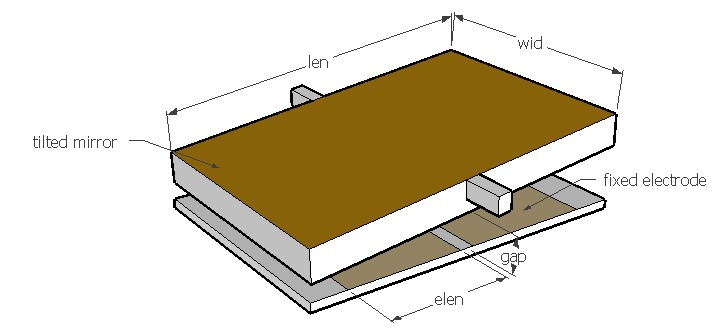TORACT,len,wid,elen,k,gap,volt,rper,sel
Torsion bar actuator for torsional motion

len         length of the plate in µm
wid         width of plate in µm
elen       length of the electrode in µm
k            torsional spring constant of the beam in µNµm/rad
gap        air gap or distance between the two plates in µm
volt        applied voltage in V
rper       relative permittivity of the medium, it is approximately 1 for air
sel         number denoting the selected result.
Use 1 for pull-in voltage, 2 for angular displacement and 3 for actuation torqueNotes

Use this design form to estimate the pull-in voltage and balanced angular displacement of an electrostatic torsion bar actuator. The actuator typically consists of a rectangular plate suspended from two torsion bars. The electrodes are placed symmetrically on the plate or below it so that voltage applied to one of the electrodes will shift the plate to one side due to electrostatic force. This driving scheme is often used in MEMS based optical mirrors. When the applied voltage is higher than the pull-in voltage, the actuator will become unstable and the plate will tilt to one side and hit the fixed electrode. This design form can be used to predict the pull-in voltage and the critical angle of the plate at the pull in voltage. Also the angular displacement at the given voltage can be predicted. The actuation torque corresponds to the balanced angular displacement and applied voltage. The maximum torque that can be generated by the actuator corresponds to the pull in voltage and critical angular displacement.

The plot shows the relation between the applied voltage and the angular displacement of the plate. The plot can be used to determine the angular displacement for any applied voltage. The maximum voltage is the pull-in voltage and the corresponding angular displacement is the critical angular displacement. After the pull-in state is reached, the plate will remain in the tilted pulled in state till the voltage is reduced below a certain level called holding voltage. That is the voltage corresponding to the maximum angular displacement shown on the graph which is at the extreme right.

Assumptions

-The electrode is assumed to extend from the middle of the plate to the distance given by the electrode length.
-The spring constant of the beam is known. If unknown, this can be calculated under Mechanics > Structures > Beams.
-The medium between the electrodes is air by default with a relative permittivity of 1.
-The gap between the electrodes is uniform when no voltage is applied.
-Damping is not considered.
-Parasitic capacitance is not considered.
-Constant driving voltage is assumed.
-There is no overshooting caused by electrostatic force.